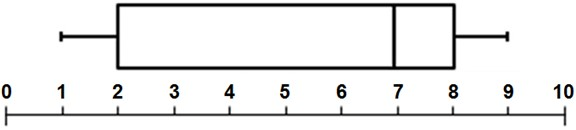© Ms. Garcia Math 7th The following box-and-whisker plot represents the scores earned on an algebra test. GR41 answersUse the box and whiser plot to answer these questions 1) What is the median ? 2) What number represents the first quartile (lower quartile)? 7 2 Answer Answer 3) What is the lower extreme? 4) What is the upper extreme? 1 9 Answer Answer 5) What number represents the third quartile (upper quartile)? 6) What number represents the second quartile? 8 7 Answer Answer Name: ID: